# OrgChart Now Help Guide

### Audience

##### Overview

An administrator can create custom formulas to display in chart boxes, on a Master Page, or in the Profile Panel.

##### Accessing the Formula Editor

Select More > Chart Settings, and then select the Fields & Formulas option from the left side menu. The Fields and Formulas panel is displayed.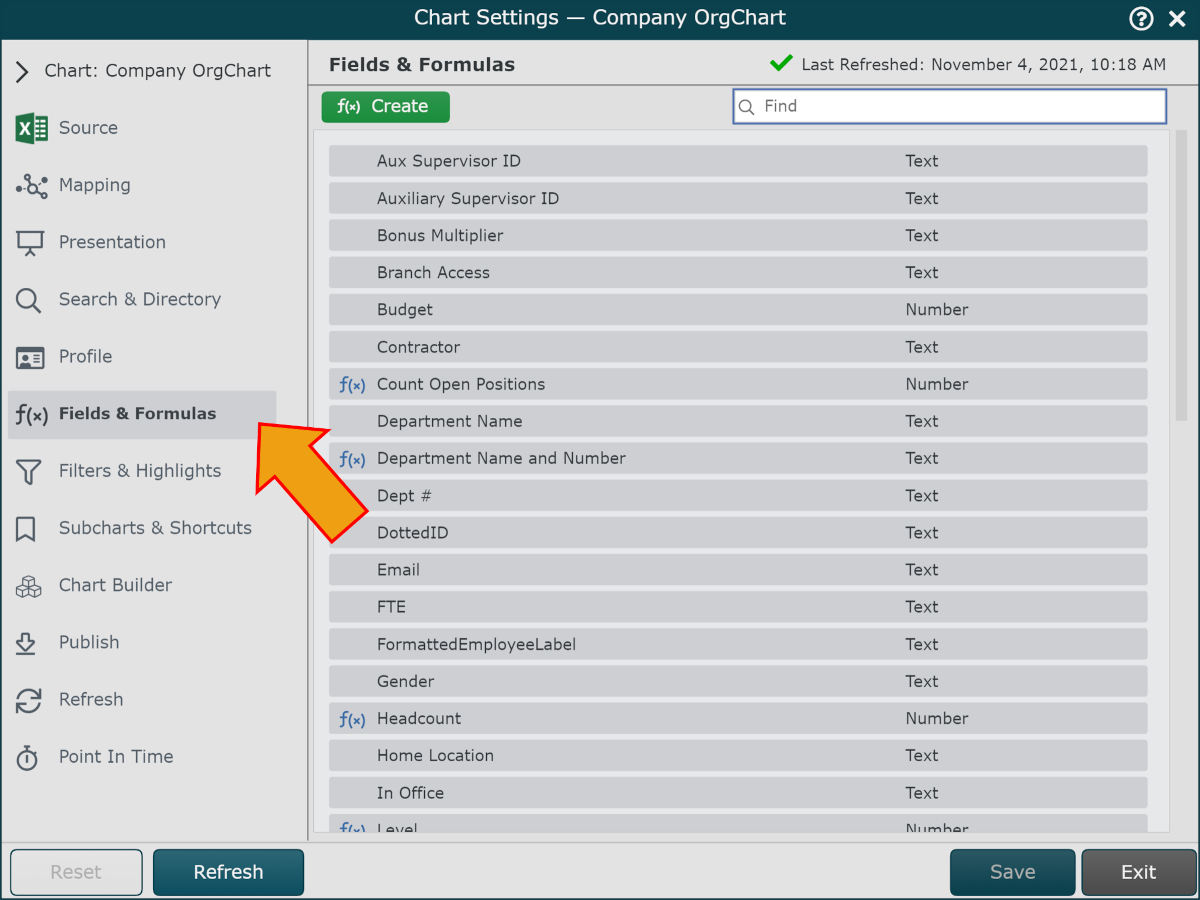The following options are available:

• Click on the + f(x) button to add a formula

• Mouse over an existing formula and then click on theto modify an existing formula

### Note

Note: Predefined formulas cannot be edited.

##### Defining a Static Formula in OrgChart Viewer

The following example demonstrates how to create a formula that counts open positions.

1. Click on the green f(x) Create button in the top left corner. The Formula Editor panel will be displayed.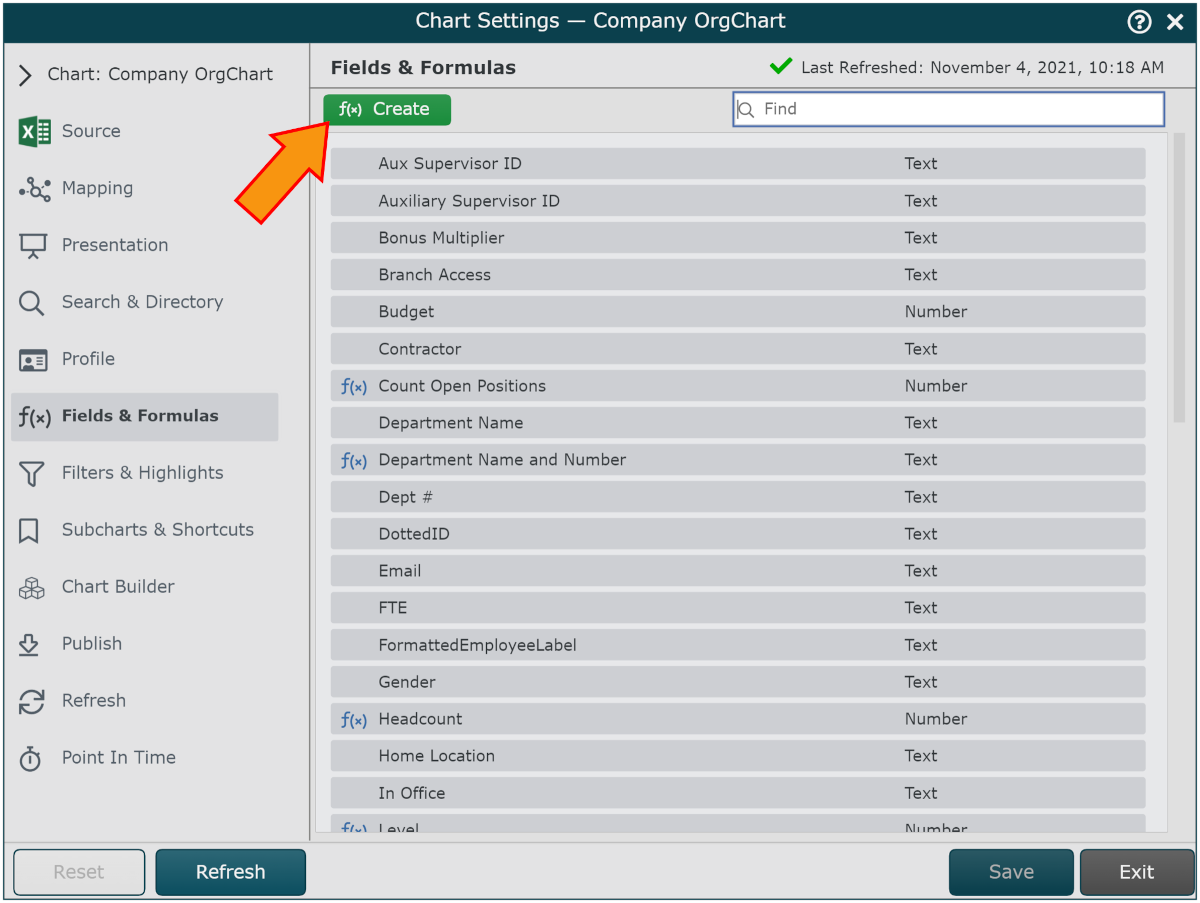2. Enter a name the new formula (e.g. Open Positions) in the Formula Name textbox.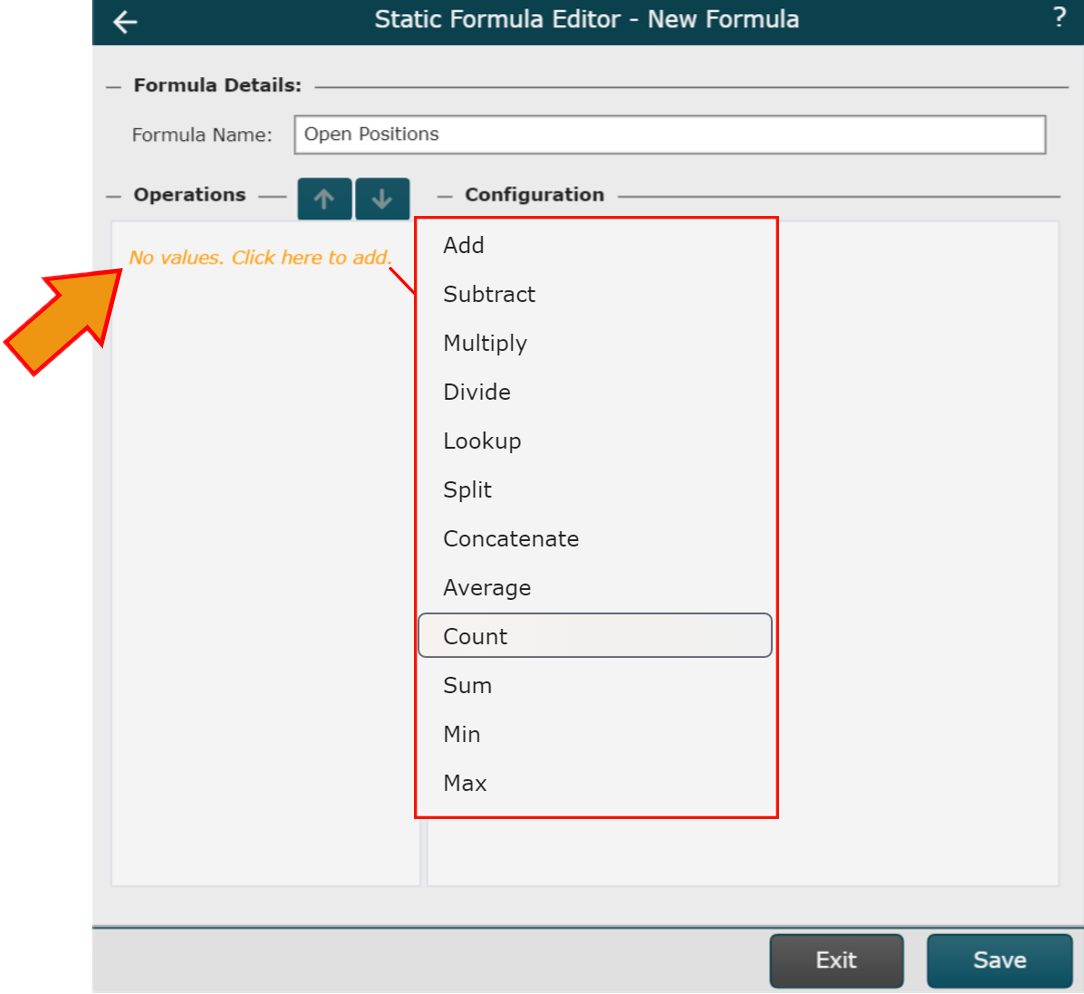4. Click on the Scope dropdown menu, and then select All Levels.

5. Check the Include Self checkbox.

6. Click on the Create Rules button.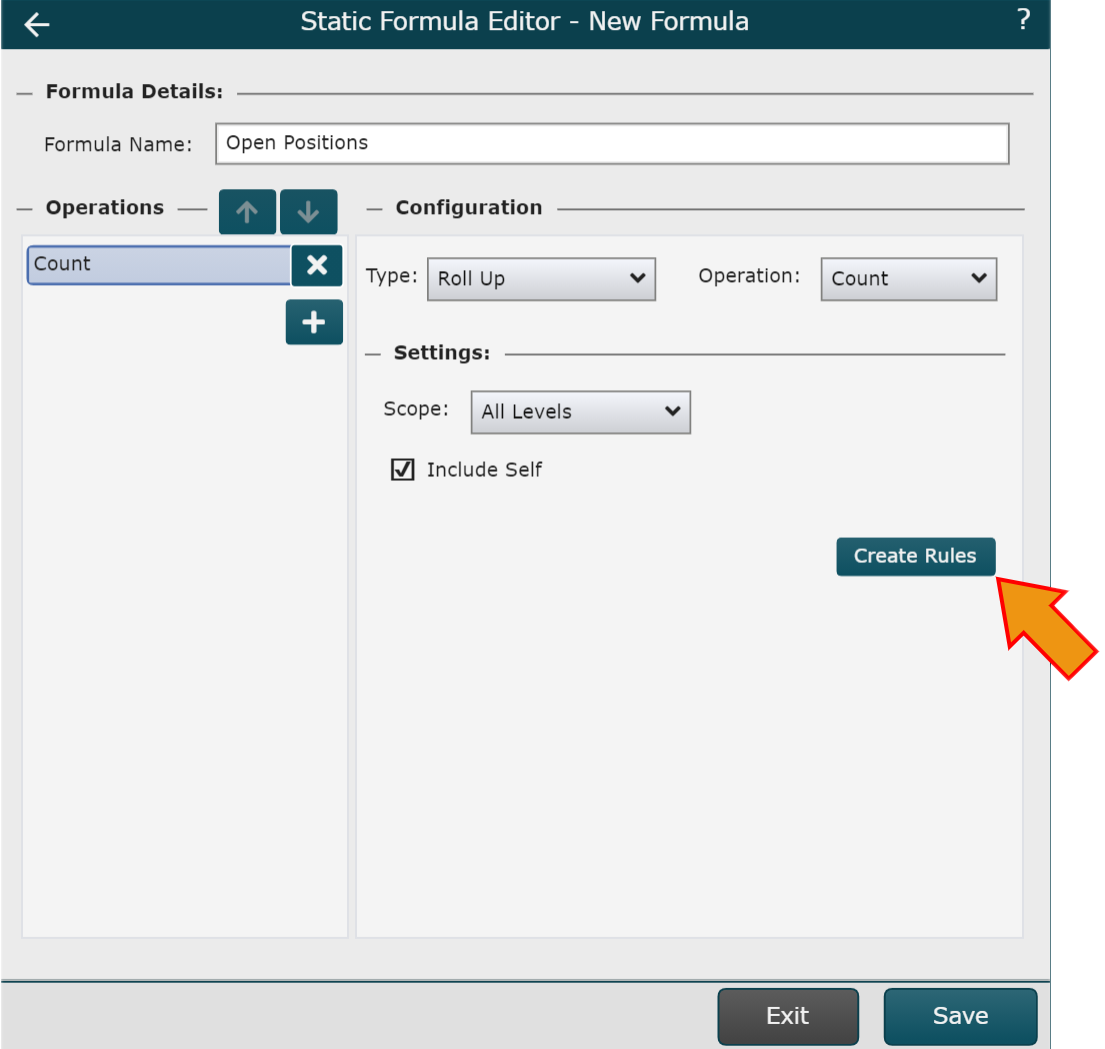7. Configure the rule as shown below. In this example, a vacancy is identified when the employee name is set to 'Vacant.' This rule definition will vary based on your source data.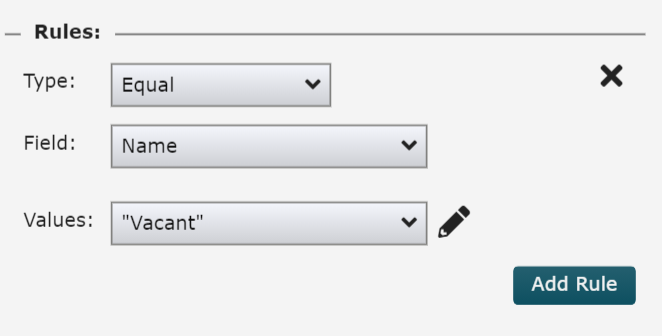8. Click Save.

##### Formatting a Formula

You can control how a formula is displayed (e.g. the number of decimal places to display). For this example, we’ll continue to work on our ‘Open Position’ count formula.

### Note

Note: Reference Field and Formula Properties section of the Fields and Formulas article for more information on available presentation options.

1. Mouse over a formula, and then click on theicon. You will be presented with formula display options.

2. Click on the Field Type dropdown, and then select the Number option. This will ensure that the formula results display as a number.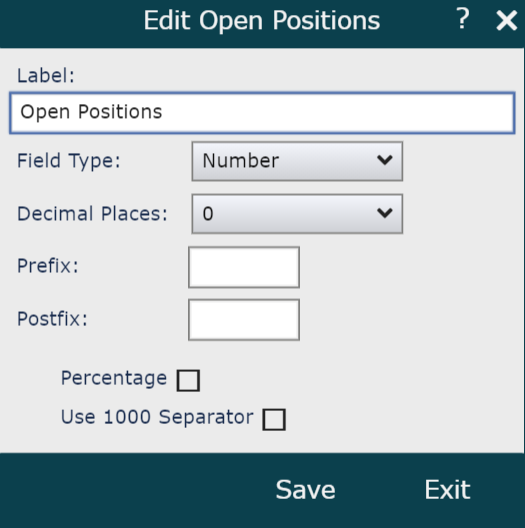3. Click Save.

##### Formula Examples

The following articles provide step-by-step instructions on how to create various types of formulas:

##### Complex Operations

You can include multiple operations in a single formula. Operations are performed top to bottom.Add an additional operationMouse over to remove an operationMove an operation upMove an operation down
##### Available Operations

Operation

Description

Subtract

Subtract the previous two values

Multiply

Multiply the previous two values

Divide

Divide the previous two values

Lookup

Lookup a record based on a field value (See example)

Split

Spilt a string based on a separator character

Concatenate

Merge two or more fields together

Average

Average a field within an org chart branch

Count

Count boxes within an org chart branch

Sum

Sum a field within an org chart branch

Max

Max value for a specified field within an org chart branch

Min

Min value for a specified field within an org chart branch